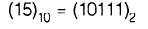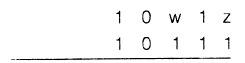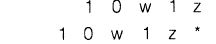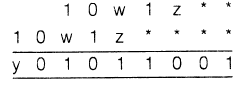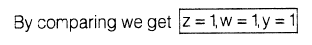Courses

# Analog & DE - MCQ Test 2

## 20 Questions MCQ Test GATE Electrical Engineering (EE) 2022 Mock Test Series | Analog & DE - MCQ Test 2

Description
This mock test of Analog & DE - MCQ Test 2 for GATE helps you for every GATE entrance exam. This contains 20 Multiple Choice Questions for GATE Analog & DE - MCQ Test 2 (mcq) to study with solutions a complete question bank. The solved questions answers in this Analog & DE - MCQ Test 2 quiz give you a good mix of easy questions and tough questions. GATE students definitely take this Analog & DE - MCQ Test 2 exercise for a better result in the exam. You can find other Analog & DE - MCQ Test 2 extra questions, long questions & short questions for GATE on EduRev as well by searching above.
QUESTION: 1

### An amplifier has an open-loop gain of 100, an input impedance of 10 kΩ and an output impedance of 200 Ω. A feedback factor of 0.99 is connected to the amplifier in a voltage series feedback configuration. The new input and output impedances respectively are

Solution: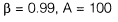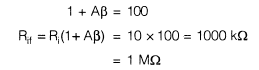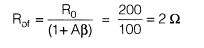QUESTION: 2

### Following shown is an oscillator circuit.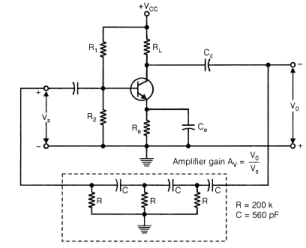Q. Which of the following is a correct statement? It is a

Solution:

It is a RC phase-shift oscillator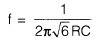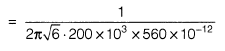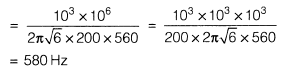QUESTION: 3

### Following shown is an oscillator circuit.Q. The gain of the amplifier must exceed _____________   to satisfy the conditions of oscillation.

Solution:

Three section RC network causes the output to be 1/29 of the input, the gain of the amplifier must exceed 29.

QUESTION: 4

Without feedback, the voltage gain is 40 and distortion is 10%, if feedback factor of -0.01 (negative feedback) is given to the amplifier, the distortion will be

Solution: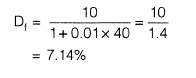QUESTION: 5

An op-amp is used in the circuit as shown in the below figure. Current l0 is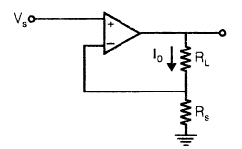Solution: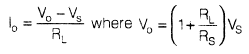QUESTION: 6

For the circuit shown in the below figure, the output F will be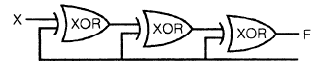Solution:

For XOR gate if both the input are same, output is zero.

QUESTION: 7

Match List-1 (Types of gates) with List-ll (Values of propagation delay) and select
the correct answer-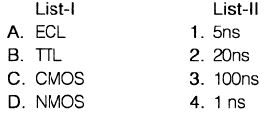Codes:
A B C D

Solution:

ECL are fastest and N-Mos devices are slowest

QUESTION: 8

The Booiean expression for the output Y in the logic circuit is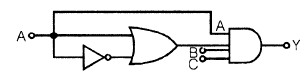Solution: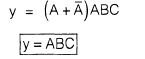QUESTION: 9

Consider the following:
Any combinational circuit can be built using
1.    NAND gates
2.    NOR gates
3.    EX - OR gates
4.    Multiplexers

Which of these are correct?

Solution:

NAND & NOR are universal gates, so along with MUX they can be used to implement any combinational circuit.

QUESTION: 10

A 3-to-8 decorder is shown below: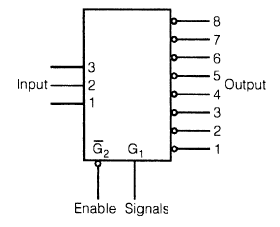All the output lines of the chip will be high, when all the inputs 1,2 and 3

Solution:

Outputs are active low, so all the output lines of the chip will be high, when chip will
be disabled i.e., G,= 0 and G2 = 1.

QUESTION: 11

The simplified form of a logic function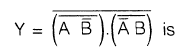Solution: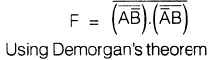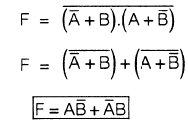QUESTION: 12

An n-bit A/D converter is required to convert analog input in the range 0 - 5V to an accuracy of 10 mV. The value of n should be

Solution:

Given minimum analog input (to be converted accurately) = 10 mV => Maximum step size should be = 10 mV as Maximum voltage = (Step size), (no. of steps)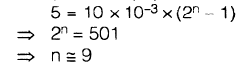QUESTION: 13

Consider the following statements in respect of ECL gate:
1.    Its switching speed is high
2.    It provides OR and NOR logic operations
3.    Its power dissipation is small as compared to other logic gates
4.    Its logic levels are compatible with other logic family gates

Which of these statements are correct?

Solution:

Its logic levels are not compatible with CMOS (15V) logic family gates.

QUESTION: 14

In the circuit as shown in figure below, assuming initially Q0 = Q1 = 0. Then the states of Q0 and Q1 immediately after the 33rd pulse are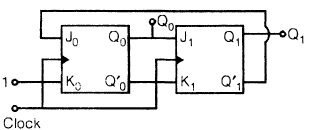Solution: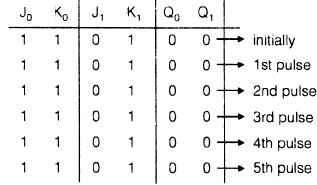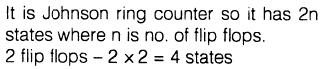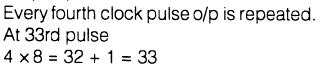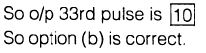QUESTION: 15

The three-stage Johnson-Ring Counter as shown below is clocked at a constant frequency of fc from the starting state of Q0 Q1Q2 = 1 0 1. The frequency of outputs Q Q1  Q2  will be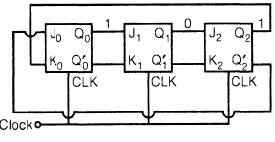Solution:

The 3 stage Johnson ring counter at initial condition 101. When CLK pulses are applied it comes into the lock out stage. So at lock out stage o/p is repeated upto n CLK pulse at which frequency of o/p isQUESTION: 16

Which one of the following statements is not correct? Conversion of EXCESS-3 code to BCD can be achieved by using

Solution:
QUESTION: 17

Match List-1 (Circuit Symbols) with List-ll (Nomenclature) and select the correct answer using the codes given below:

List-1    List-ll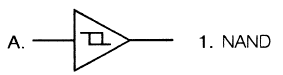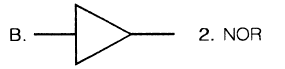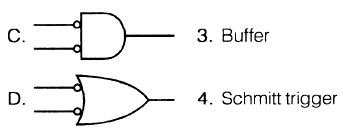Codes:
A B C D

Solution:
QUESTION: 18

It is required to construct a 2n-to-1 multiplexer by using 2-to-1 multiplexers only. How many of 2-to-1 multiplexers are needed?

Solution:
QUESTION: 19

Consider the following circuit: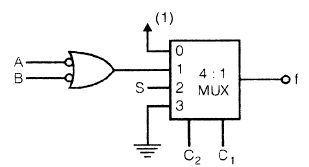Which one of the following gives the function implemented by the MUX-based diaital circuit?

Solution: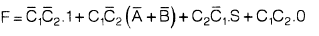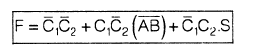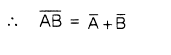QUESTION: 20

Consider the following multiplication: (10w 1z)2 x(15)10 = (y0 10 11 001)2 Which one of the following gives appropriate values of w, y and z?

Solution: